Courses

# NCERT Solutions Chapter 12 - Heron's Formula (I), Class 9, Maths Class 9 Notes | EduRev

Created by: Indu Gupta

## Class 9 : NCERT Solutions Chapter 12 - Heron's Formula (I), Class 9, Maths Class 9 Notes | EduRev

The document NCERT Solutions Chapter 12 - Heron's Formula (I), Class 9, Maths Class 9 Notes | EduRev is a part of Class 9 category.
All you need of Class 9 at this link: Class 9

1. A traffic signal board, indicating 'SCHOOL AHEAD', is an equilateral triangle with side 'a'. Find the area of the signal board, using Heron’s formula. If its perimeter is 180 cm, what will be the area of the signal board?

Length of the side of equilateral triangle = a
Perimeter of the signal board = 3a = 180 cm
∴ 3a = 180 cm ⇒ a = 60 cm
Semi perimeter of the signal board (s) = 3a/2
Using heron's formula,
Area of the signal board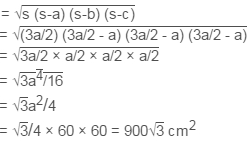2. The triangular side walls of a flyover have been used for advertisements. The sides of the walls are 122 m, 22 m and 120 m (see Fig. 12.9). The advertisements yield an earning of ₹5000 per m2 per year. A company hired one of its walls for 3 months. How much rent did it pay?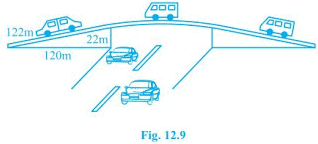The sides of the triangle are 122 m, 22 m and 120 m.
Perimeter of the triangle is 122 + 22 + 120 = 264m
Semi perimeter of triangle (s) = 264/2 = 132 m
Using heron's formula,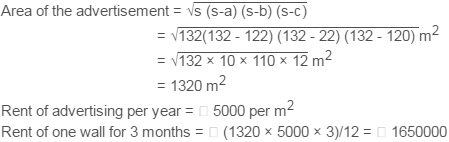3. There is a slide in a park. One of its side walls has been painted in some colour with a message “KEEP THE PARK GREEN AND CLEAN” (see Fig. 12.10 ). If the sides of the wall are 15 m, 11 m and 6 m, find the area painted in colour.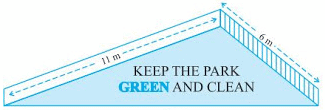Sides of the triangular wall are 15 m, 11 m and 6 m.
Semi perimeter of triangular wall (s) = (15 + 11 + 6)/2 m = 16 m
Using heron's formula,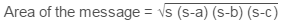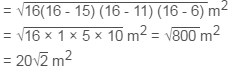4. Find the area of a triangle two sides of which are 18cm and 10cm and the perimeter is 42cm.

Two sides of the triangle = 18cm and 10cm
Perimeter of the triangle = 42cm
Third side of triangle = 42 - (18+10) cm = 14cm
Semi perimeter of triangle = 42/2 = 21cm
Using heron's formula,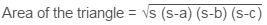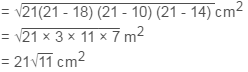5. Sides of a triangle are in the ratio of 12 : 17 : 25 and its perimeter is 540cm. Find its area.

Ratio of the sides of the triangle = 12 : 17 : 25
Let the common ratio be x then sides are 12x, 17x and 25x
Perimeter of the triangle = 540cm
12x + 17x + 25x = 540 cm
⇒ 54x = 540cm
⇒ x = 10
Sides of triangle are,
12x = 12 × 10 = 120cm
17x = 17 × 10 = 170cm
25x = 25 × 10 = 250cm
Semi perimeter of triangle(s) = 540/2 = 270cm
Using heron's formula,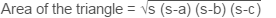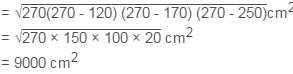6. An isosceles triangle has perimeter 30 cm and each of the equal sides is 12 cm. Find the area of the triangle.

Length of the equal sides = 12cm
Perimeter of the triangle = 30cm
Length of the third side = 30 - (12+12) cm = 6cm
Semi perimeter of the triangle(s) = 30/2 cm = 15cm
Using heron's formula,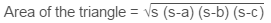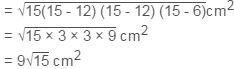,

,

,

,

,

,

,

,

,

,

,

,

,

,

,

,

,

,

,

,

,

,

,

,

,

,

,

,

,

,

,

,

;